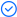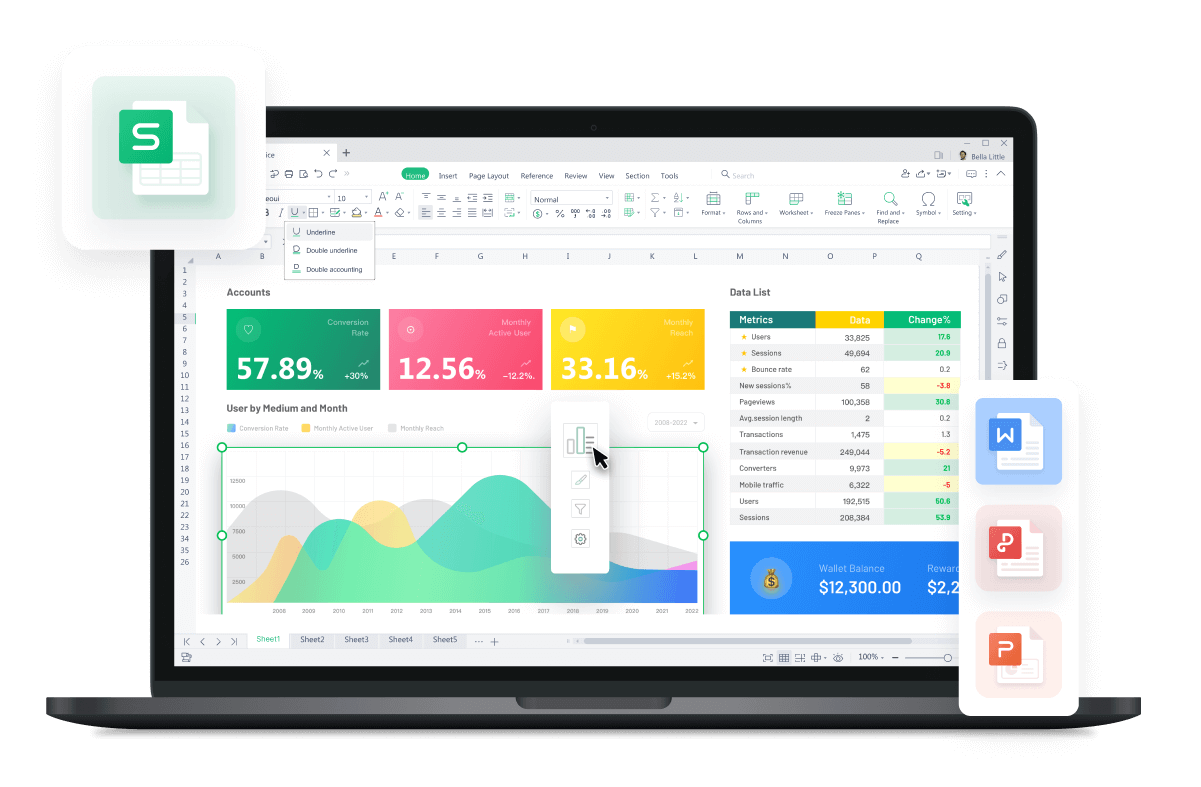WPS Office

Free All-in-One Office Suite with PDF EditorRead, edit, and convert PDFs with the powerful PDF toolkit.Microsoft-like interface, easy to use.

Windows • MacOS • Linux • iOS • Android# How to use IF function in WPS excel

August 1, 2022
4.3K Views

One of the most used functions in Excel is the IF function, which enables you to compare values logically to expectations. Thus, an IF statement can produce two outcomes. We use the IF function (a logical function) to return one value if a condition is true and the other is false.

The syntax of IF function is:

=IF (logical_test, [value_if_true], [value_if_false])

When using the IF function to construct a test, we can use the following logical operators:

= (equal to)

> (greater than)

>= (greater than or equal to)

(not equal to)

Methods related to IF function that are provided in this article are compatible with version 2016/2019/mac/online.

To Calculate Depreciation On Different Assets

1. To calculate for example the depreciation rate on different assets (text), you can use the IF formula. Here we will use = (equal to).

2. Type =IF in the target cell and double-click.

3. We have given the condition in the argument that if the nature is ‘furniture’, the amount will be depreciated by 20 percent.

4. Press enter to see result.

5. Consequently, the conditions change as you move downwards.

6. If it is too tedious to type in the formula in a new cell again and again, the formula can be summarized in the following pattern in one cell. Note that the “0” is placed only once at the end and the closing brackets are equal to the number if time IF function is used. Here, text is wrapped to show a compact formula.

7. To copy this formula in the remaining column's cells, just click twice on the fill handle at the bottom-right corner of each cell or hold and drag it down. Or use Ctrl+C (copy) and Ctrl+V (paste) on keyboard for shorter lists.

# To Mark Pass And Fail In A Students’ Result

1. If you are a teacher and you are looking for a function to mark Pass and Fail, the IF function can be very helpful.

2. Enter =IF in the target cell.

3. Double click on the IF function and type in the argument. Let the passing score be 40. The formula states, If the value in C6 is less than 70, return Fail. Otherwise, return Pass. We will use Greater Than or Equal To (>=) here.

4. To duplicate this formula in the remaining column's cells, just click twice on the fill handle at the bottom-right corner of each cell or hold and drag it down. You can also copy the formula using Ctrl+C on your keyboard and paste using Ctrl+V onto the whole column. The formula would be changed according to corresponding row automatically. The result is satisfactory.

To State Whether An Amount Meets A Certain Condition

1. If we have the following data, we can use IF function to first calculate the net rate with a specific discount.

2. Double-click on the IF function after typing =IF in target cell.

3. We have applied the condition in the argument that if the rate is less than or qual to 15, display the same rate or else display the value with a discount.

4. Press enter to see result and copy paste the formula in the remaining cells.

5. Now, if we need to find a certain text condition, for example whether the net rate is greater than 10 or no, we can use IF function for text here as well. We have applied the condition that if the net rate is greater than 10, display Yes, otherwise No.

6. Press enter.

7. To duplicate this formula in the remaining column's cells, just click twice on the fill handle at the bottom-right corner of each cell or hold and drag it down. Or select the text cell with the formula and press Ctrl+C for copying and Ctrl+V for pasting it onto the cell below. Continue till all the cells have been filled.